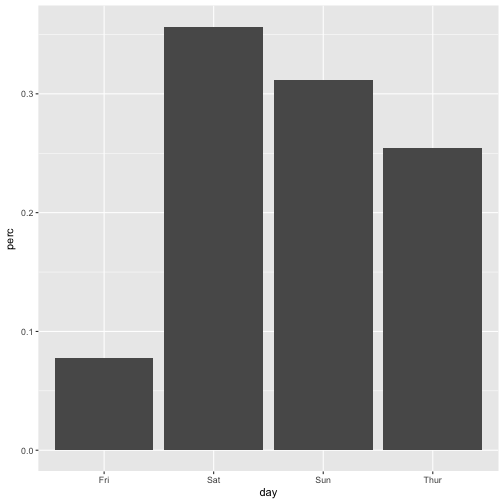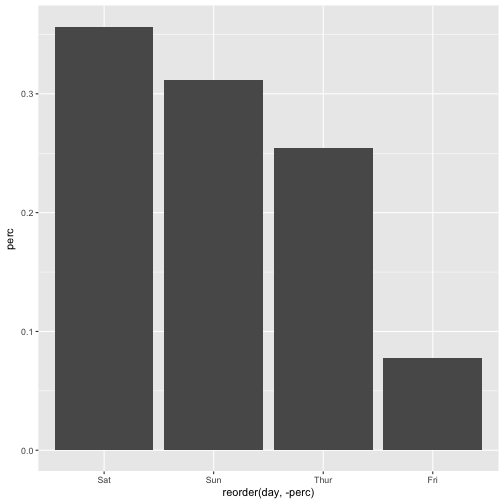# Sorting the x-axis in bargraphs using ggplot2

## June 05, 2017

Some time ago, I posted about how to plot frequencies using ggplot2. One point that remained untouched was how to sort the order of the bars. Let’s look at that issue here.

data(tips, package = "reshape2")


And the usual culprits.

library(tidyverse)
library(scales)  # for percentage scales


First, let’s plot a standard plot, with bars unsorted.

tips %>%
count(day) %>%
mutate(perc = n / nrow(tips)) -> tips2

ggplot(tips2, aes(x = day, y = perc)) + geom_bar(stat = "identity")Hang on, what could ‘unsorted’ possibly mean? There must be some rule, by which ggplot2 determines order.

And the rule is:

• if factor, the order of factor levels is used
• if character, an alphabetical order ist used

# Sorting bars by factor ordering

Albeit it appears common not to like factors, now that’s a situation when they are useful. Factors provide an easy for sorting, see:

tips2$day <- factor(tips2$day,levels = c("Fri", "Sat", "Sun", "Thur"))


Now let’s plot again:

ggplot(tips2, aes(x = day, y = perc)) + geom_bar(stat = "identity")# Sorting bars by some numeric variable

Often, we do not want just some ordering, we want to order by frequency, the most frequent bar coming first. This can be achieved in this way.

ggplot(tips2, aes(x = reorder(day, -perc), y = perc)) + geom_bar(stat = "identity")Note the minus sign -, leaving it out will change the ordering (from low to high).

Happy plotting!